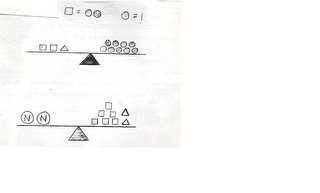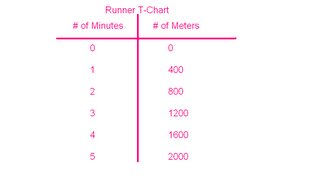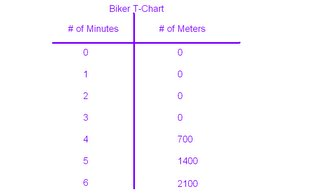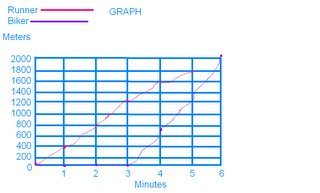## Saturday, May 27, 2006

### My Growing Post Due Monday May 29th

Question 1: What is the difference between an algebraic expression and an equation (hint: One contains this and the other doesn't)? Give an example of both an expression and an equation.

In an algebraic expression you get to choose the value of the variable.
In an algebraic equation the value of the variable is given.
Algebraic Expression: 9N+4
Algebraic Equation: 9N+4=22

Question 2: What is a variable and why do we use one in algebra?

A variable is a letter that replaces a number. We use a variable in algebra because algebra is about solving the uknown and the variable is the unknown thing we have to solve.

Question 3: Solve for NTo solve the second question, you need to figure out what a triangle equals on the first scale.
First, I took away four circles (each square equals two circles), which left me with five circles. Since there were five circles left, the triangle equals five circles.
To solve the second question, you need to figure out the value of the two Ns.
First, I added the squares and triangles together. The total of the squares and triangles, together, was 22 circles. Then I divided the 22 circles by 2. The answer was 11. So each N equals 11 circles.

Question 4: Solve the following equations. Show all of the steps that are needed.

(a) 3n+4n+7=2n+12

7n+7=2n+12

7n-2n(+7-7)=2n-2n(+12-7)

5n=5

n=1

(b) 8n-(4+9)=11

8n-13=11

+13 +13

8n=24

n=24/8

n=3

(c) (8-3)n+7n+8=4n+40

5n+7n+8 =4n+40

12n+8=4n+40

12n(+8-8)=4n(+40-8)

12n-4n=(4n-4n)+32

8n=32

n=4

Question 5: For this question you need to create a T-Chart and a graph to plot your data from this question onto.You are having a race against your friend, except you are on foot and he is on his bike. Your both know that if you are on a bike you will be faster so your friend gives you a head start of 3 minutes. If you can run 400m per minute, and your friend can bike 700m per minute, who will be the first to make it to the finish line 2000m away?

The runner will cross the finish line first in 5 minutes. The biker will cross the finish line second in 6 minutes.

Kendra Valentonis 7-43

At 5/27/2006 9:43 PM,Aria said...

X3 Nice lines Fang. Good job!

At 5/28/2006 3:19 PM,Candy said...

This comment has been removed by a blog administrator.

At 5/29/2006 2:02 PM,Fang_the_Barbarian said...

candace my name is kendra not kendar

At 5/29/2006 3:13 PM,Smiiliies said...

Good Job Kendra, I really like yours.

Kim

At 5/29/2006 3:15 PM,Candy said...

Great Job Kendra !!!!!!!!!!!!!!!!

At 5/30/2006 9:33 AM,amelia_ mal said...

Hey Kendra! great job you must have put alot of time on it.
it's Amalia

At 5/30/2006 3:37 PM,Fang_the_Barbarian said...

THANK YOU EVERYONE FOR THE COMMENTS!

At 6/01/2006 4:36 PM,Mr. Reece said...

Is the value of the variable given, or is the solution to the equation given, and using the solution we can find the exact value of the variable?

I think that we were to solve for n using numbers not shapes, but that shouldn't be hard for you to change.
Otherwise great job

At 6/19/2006 2:39 PM,Mr. Reece said...